# I Drew a Tesseract (Without even knowing how)

We all know that a tesseract (hypercube) is a 4D object analogous to a 3D cube or a 2D square. I decided that I wanted to draw one, despite the fact that I have no idea how. I did know, however, that an edge of a cube is a set of points in which two out of the three spatial coordinates are extremal (relative to the cube's size), and one can vary continuously between the extremes.

What if we did the same thing for a 4D hypercube? Create regions of points where three out of the four spatial dimensions are at extreme values, and only one can vary continously. I wrote a program based on the following processing:

1) Conceptualize a 4D coordinate system (x,y,z,w)

2) Randomly generate a set of four 4D orthogonal basis vectors. These vectors make the plots more interesting than they presumably would be if we used the simple (1,0,0,0), (0,1,0,0), (0,0,1,0), (0,0,0,1) set. See the attached code for these vectors.

3) Each plotted point is a weighted sum of the vectors from Step 2, where each basis vector has its own weight

4) Randomly generate the weights so that three of them are at extreme values (either +1 or -1), and the remaining weight varies continuously between -1 and +1. This gives us points along the edges of the 4D hypercube.

5) Make scatter plots with two of the four dimensions. Plotted combinations are xy, xz, xw, yz, yw, zw.

Plots and code are below. I think the first and the sixth plots are the coolest to look at. These are 2D projections of 4D hypercubes, generated purely from randomly generated points. They are not super high-res because I didn't want to put too many points into Excel.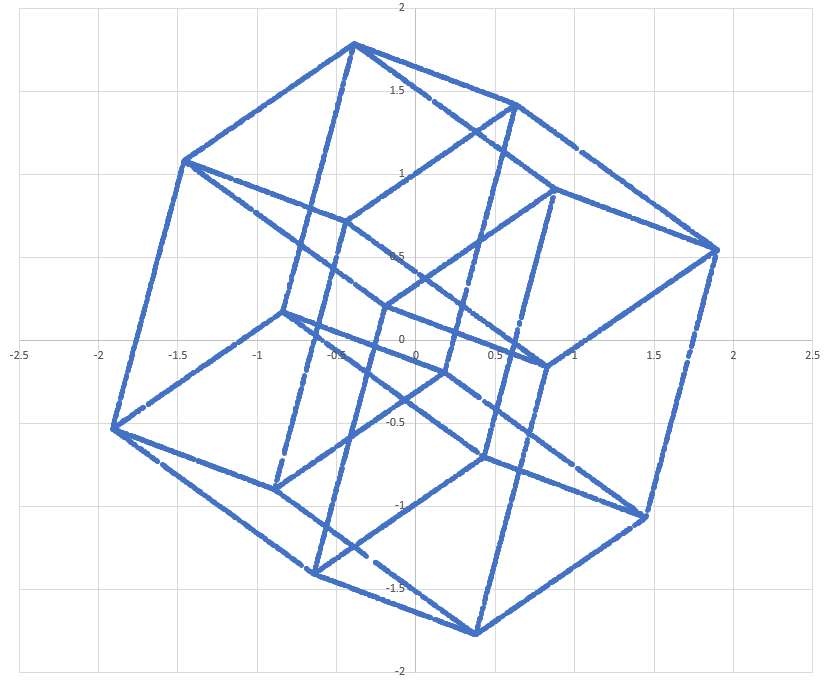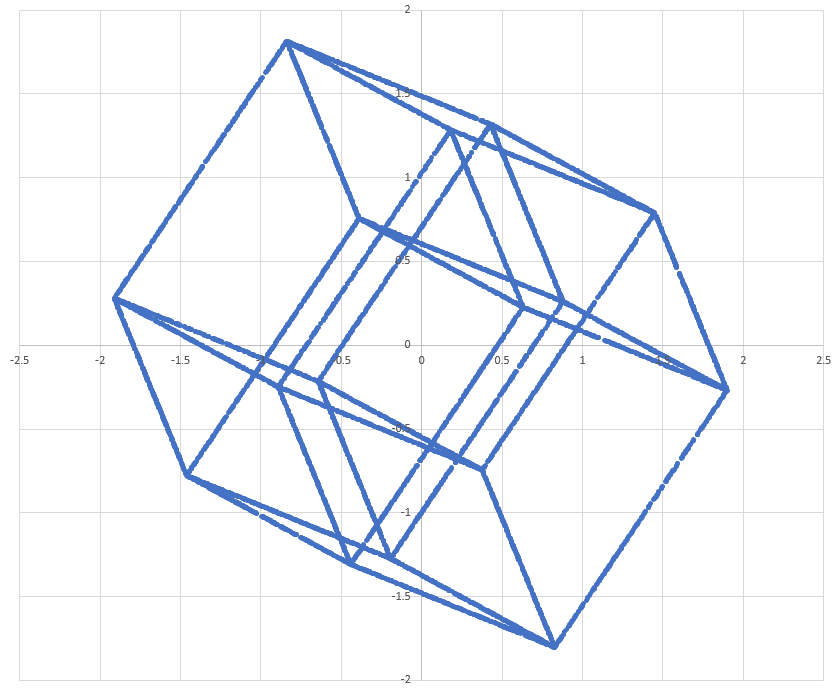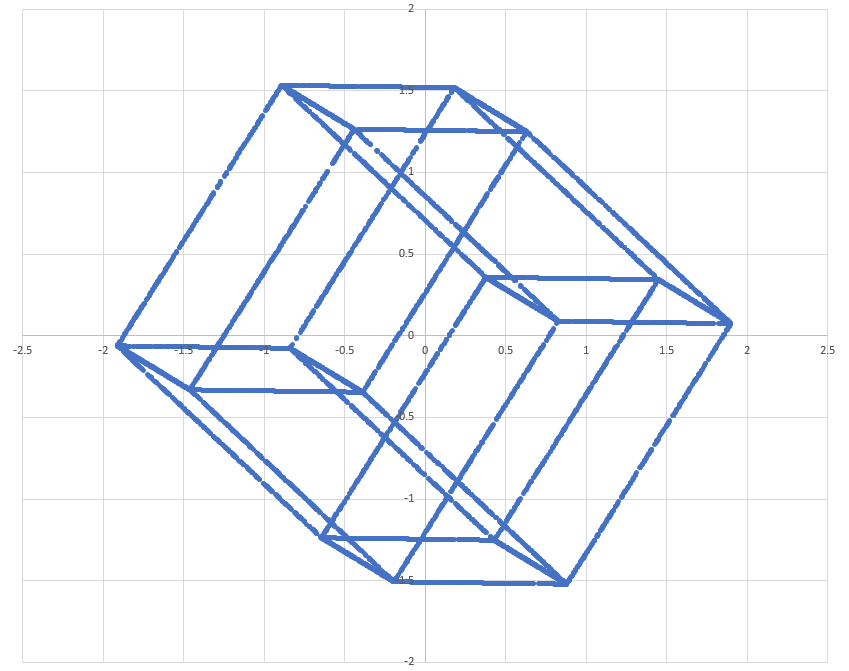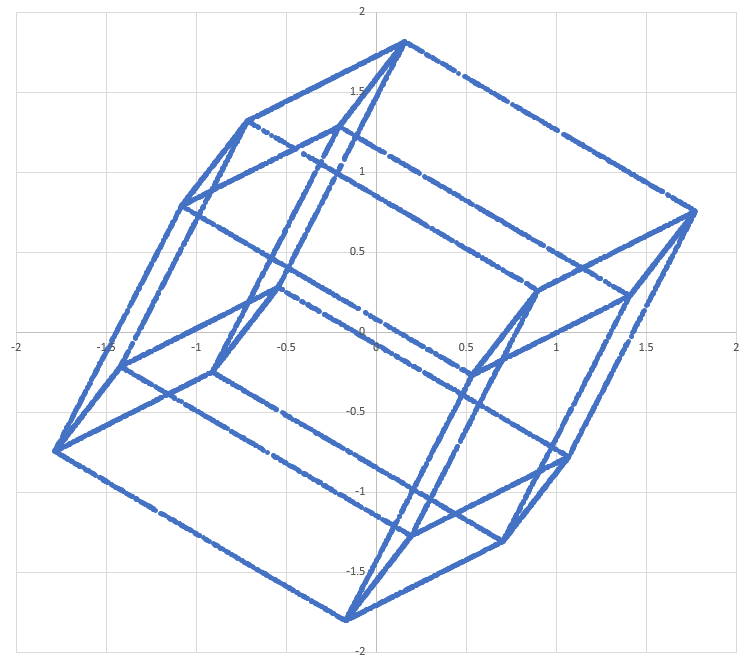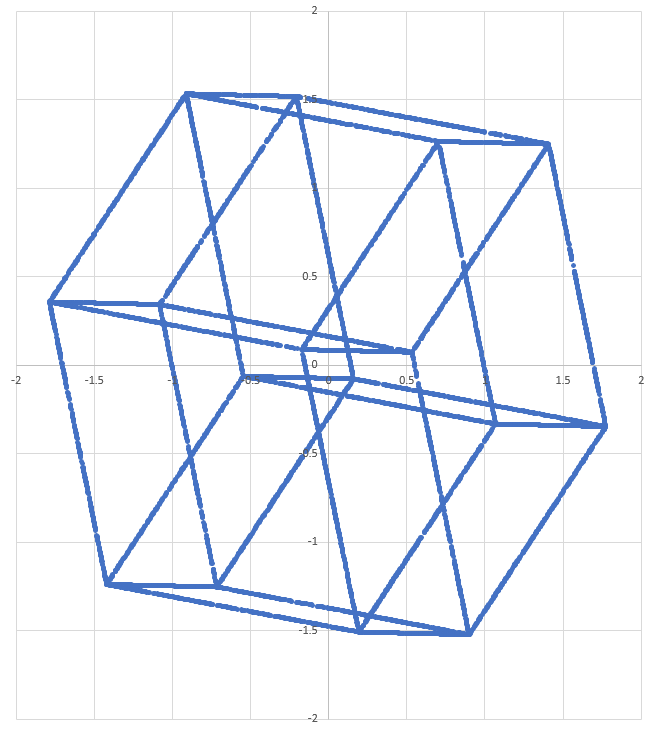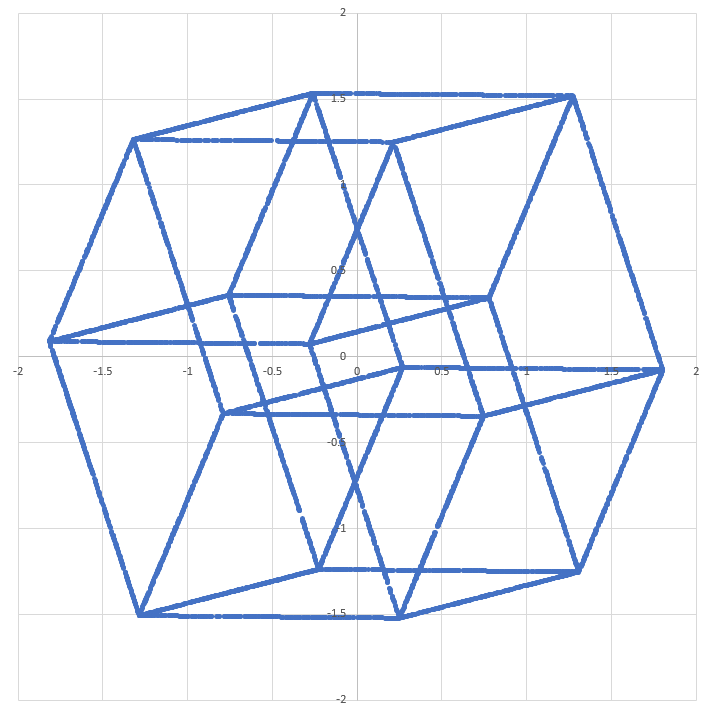1 2 3 4 5 6 7 8 9 10 11 12 13 14 15 16 17 18 19 20 21 22 23 24 25 26 27 28 29 30 31 32 33 34 35 36 37 38 39 40 41 42 43 44 45 46 47 48 49 50 51 52 53 54 55 56 57 58 59 60 61 62 63 64 65 66 67 68 69 70 import math import random sx = 0.509967921254 sy = -0.18278826241 sz = -0.264779918013 sw = 0.797754978662 tx = 0.536030522728 ty = 0.352271346186 tz = 0.767147895871 tw = -0.00734503318408 ux = 0.225111754835 uy = 0.806999040406 uz = -0.529133775059 uw = -0.134618545946 vx = 0.633989716835 vy = -0.437354662725 vz = -0.247769765951 vw = -0.587716669068 for j in range(0,10**4): var = random.randint(1,4) if var == 1: s = random.uniform(-1.0,1.0) t = (-1.0)**(random.randint(0,1)) u = (-1.0)**(random.randint(0,1)) v = (-1.0)**(random.randint(0,1)) if var == 2: t = random.uniform(-1.0,1.0) s = (-1.0)**(random.randint(0,1)) u = (-1.0)**(random.randint(0,1)) v = (-1.0)**(random.randint(0,1)) if var == 3: u = random.uniform(-1.0,1.0) s = (-1.0)**(random.randint(0,1)) t = (-1.0)**(random.randint(0,1)) v = (-1.0)**(random.randint(0,1)) if var == 4: v = random.uniform(-1.0,1.0) s = (-1.0)**(random.randint(0,1)) t = (-1.0)**(random.randint(0,1)) u = (-1.0)**(random.randint(0,1)) x = s*sx + t*tx + u*ux + v*vx y = s*sy + t*ty + u*uy + v*vy z = s*sz + t*tz + u*uz + v*vz w = s*sw + t*tw + u*uw + v*vw #print x,y #print x,z #print x,w #print y,z #print y,w print z,w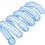Note by Steven Chase
2 years, 6 months ago

This discussion board is a place to discuss our Daily Challenges and the math and science related to those challenges. Explanations are more than just a solution — they should explain the steps and thinking strategies that you used to obtain the solution. Comments should further the discussion of math and science.

When posting on Brilliant:

• Use the emojis to react to an explanation, whether you're congratulating a job well done , or just really confused .
• Ask specific questions about the challenge or the steps in somebody's explanation. Well-posed questions can add a lot to the discussion, but posting "I don't understand!" doesn't help anyone.
• Try to contribute something new to the discussion, whether it is an extension, generalization or other idea related to the challenge.

MarkdownAppears as
*italics* or _italics_ italics
**bold** or __bold__ bold
- bulleted- list
• bulleted
• list
1. numbered2. list
1. numbered
2. list
Note: you must add a full line of space before and after lists for them to show up correctly
paragraph 1paragraph 2

paragraph 1

paragraph 2

[example link](https://brilliant.org)example link
> This is a quote
This is a quote
    # I indented these lines
# 4 spaces, and now they show
# up as a code block.

print "hello world"
# I indented these lines
# 4 spaces, and now they show
# up as a code block.

print "hello world"
MathAppears as
Remember to wrap math in $$ ... $$ or $ ... $ to ensure proper formatting.
2 \times 3 $2 \times 3$
2^{34} $2^{34}$
a_{i-1} $a_{i-1}$
\frac{2}{3} $\frac{2}{3}$
\sqrt{2} $\sqrt{2}$
\sum_{i=1}^3 $\sum_{i=1}^3$
\sin \theta $\sin \theta$
\boxed{123} $\boxed{123}$

Sort by:

The last picture is really the best! I think it's like a stack of 2 cubes where the middle layer is pushed outside a little bit to make parallelograms as sides. Then, this part is copied, rotated 90° around the vertical axis and the top and bottom square of the two copies are aligned. Thank you for giving me a new understanding of the four dimensional world!

- 2 years, 6 months ago

Yeah, I agree. Two cubes with connected vertices, and different orientations of that within the same object, with no contradictions. Thanks for commenting, and Happy New Year

- 2 years, 6 months ago